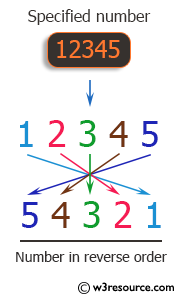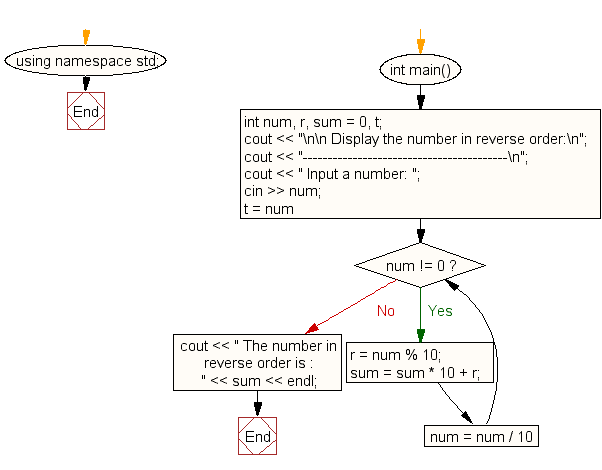﻿ C++ Exercises: Display the number in reverse order - w3resource

# C++ Exercises: Display the number in reverse order

## C++ For Loop: Exercise-30 with Solution

Write a program in C++ to display the numbers in reverse order.

Pictorial Presentation:Sample Solution:-

C++ Code :

``````#include <iostream>
using namespace std;

int main()
{
int num, r, sum = 0, t;
cout << "\n\n Display the number in reverse order:\n";
cout << "-----------------------------------------\n";
cout << " Input a number: ";
cin >> num;
for (t = num; num != 0; num = num / 10)
{
r = num % 10;
sum = sum * 10 + r;
}
cout << " The number in reverse order is : " << sum << endl;
}
``````

Sample Output:

``` Display the number in reverse order:
-----------------------------------------
Input a number: 12345
The number in reverse order is : 54321
```

Flowchart:C++ Code Editor: